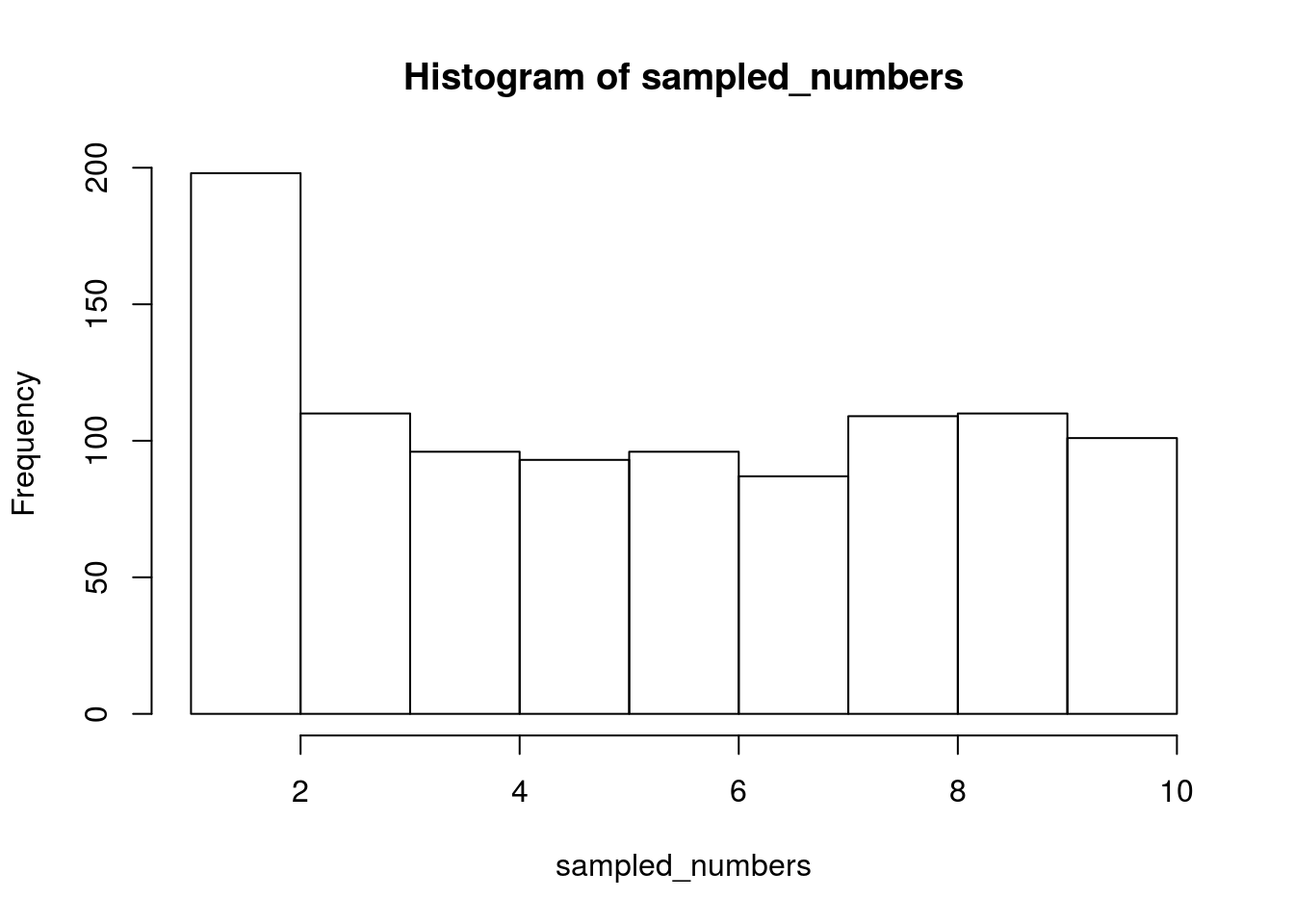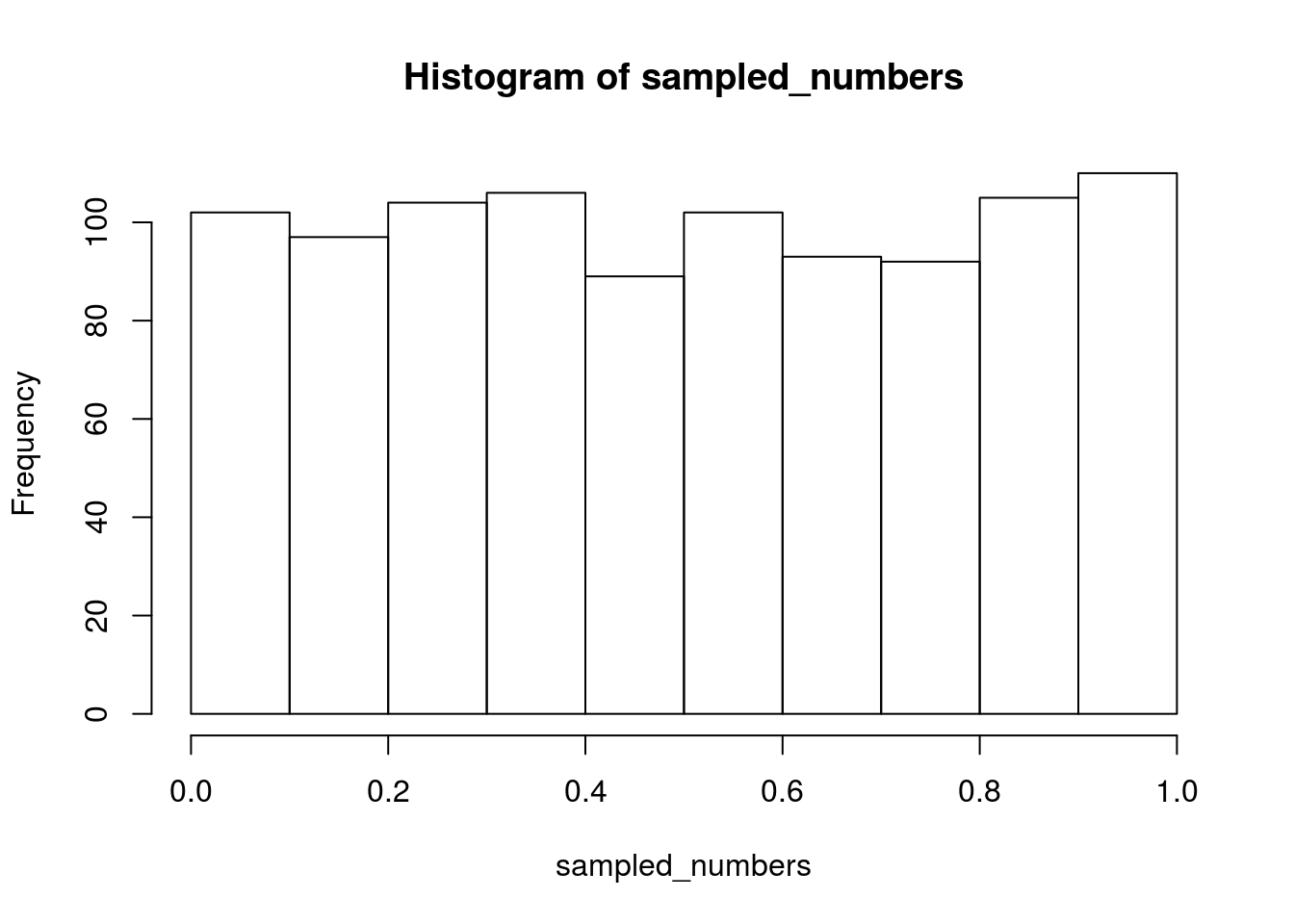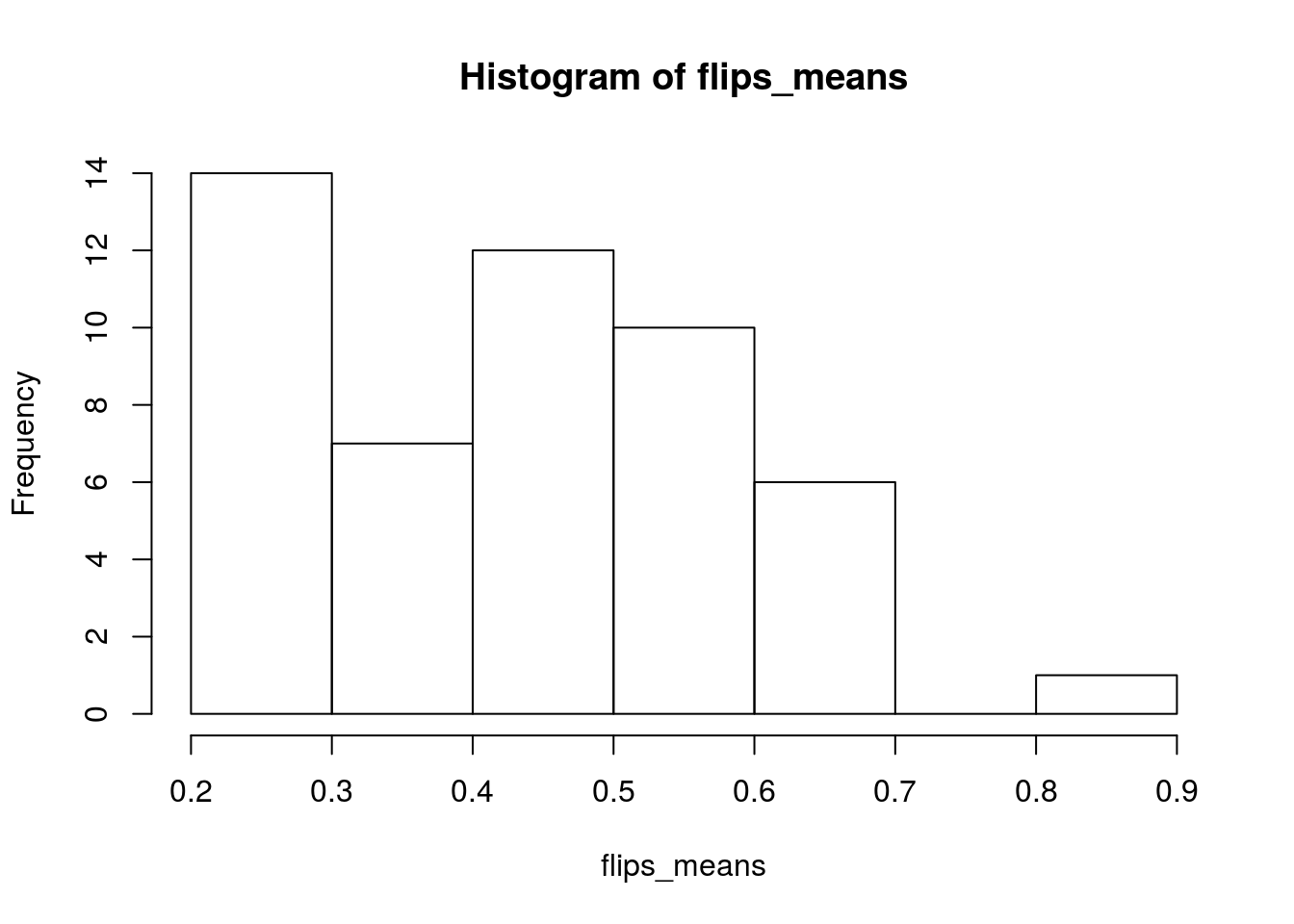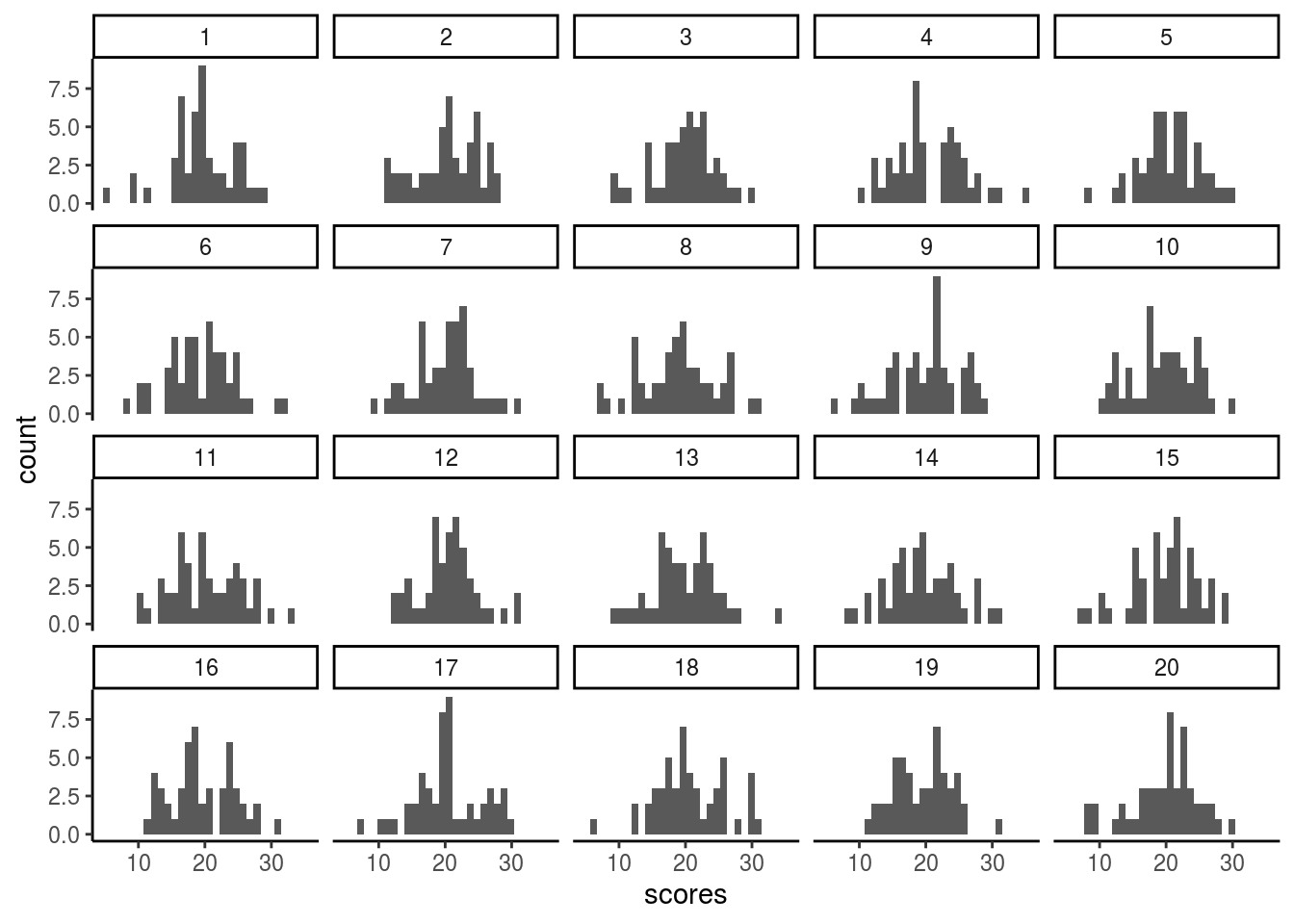Today we’ll look at ways of how to generate random numbers in R and we’ll also do some very simple statistical simulations.

# sample

First tool we’ll use to generate random numbers is sample. Random sampling is a key tool that allows us to move from the descriptive statistics to the inferential statistics. In R, we can simply use sample to select a subset from a population.

numbers <- c(1,2,3,4,5,6,7,8,9,10)
sampled_numbers <- sample(numbers, 1000, replace=TRUE)
sampled_numbers
##      3 10  6  5  3  4  2  6  4  8  4 10  3  5  6  9  2  5  1  3  2  4  3
##     2  2  9  9  6 10  4  1  7 10  6  2  3  3  5  2  7  7 10 10 10 10  3
##     8  6  4  4  2  2 10  3  5  3  8  2  4  8  2  4  3  1 10  7  3  1  8
##     4  7 10  4  8  5  7  5  6  1  2  5  7  3  5  9  6  4  6  4  4  8  5
##     2  5  2  6  9  3  4 10  6  1  6  7 10  6 10  6  4  1  8  5  2  4  2
##    2 10 10  8  5  6  3 10 10 10  9  4  7 10 10  8  9  2  7  7  9 10  3
##    8  2  8 10  6  6  8  4 10  7  2  5  1  5  9  5  3  5 10  6  1  6  9
##    2  2  3  1  7  7  1  8  3  3  2  4 10  8  7 10  3  9  2  4  6  5  6
##    8  6  6 10  2  4  1  7  7  2  2  7  2  8  2  1  1  8  2  9  1  3  8
##   10  7  1  9  9  5 10  9  7  9  7  4 10  9  3  1  2  8  5  5  1  8  8
##    2 10  2  7  5 10  3  1  1  6  6  6  8  2  1  2  2  1  4  7  5  3  8
##   10  4  2  7  3  6  8  6  8  8 10  6  9  4  9  7  3  9  1  4  7  9  9
##    1 10  2  7  5  4  3  7  8  5  4  2  3  7  3  4  2  6  8  6  2  8  9
##    8  5 10  9  6  3  9  5  3  8  1  9  6  5  4  3  9 10  3  6  2  8  1
##    2  2  9  7  2  2 10  8  3  6  9  8  6  9  5  8  3  1  7  9  8  4  3
##    6  6  3  6  9  1  4  8  9  5 10  8  1  5  9  3  6  2 10  7  7  1  7
##    3  6  3  3  8  6  8 10  9  9  8  7  9  1  1  7  5  9  9  8  7  9  5
##   10  4 10  2  7  1  7  8  4  1  4 10  2  9  1  7  3  4  5 10  9  1  7
##    3  3  3  1  5  6  4  3  2  7  3  1  8  3  2  9  2  4  3  8  1  1  2
##    9  2  6  6  8  9  6  1  6  4  3  3 10 10  8  2  9  7  5  2  2  2  5
##    1  8  6  8  5  9  1 10  6  1 10 10 10  2  9  7  4  1  4  4  3  3  8
##    5 10  6 10 10  8  5  6  9  3 10  7  5  1  3 10  2  5 10  3  9  9  4
##    9  3  6  5  9  8  3  1  8  5  6  4  3  3  8  2  4  2  9  1  8  9 10
##    7  1  5  9  3  6  4  7  8  2  6  4  9  9  2  7  9  6  8  5  9  4  6
##    2  7  5  5  1  1  4  6  4  3  4  3  3  4  5  5  8  4  5  2  4  3  9
##    2  7  5  5  7  7  2  1 10  5  6  1  3  8  2  3  5  9 10  4  3  8  6
##    4  8 10  9  1  6  3  7  3  5  6  9  9 10  2  6  6  8  6  7  1  7  2
##    2  8  6  4  3  5  7  3  4  4  3  4  6  2  2  9  9  4  9 10  8  4  1
##    7  8 10  9  2  8  8  6  4 10  9 10  7  1  7  6  8  2  4  6  1  4  3
##    8  8  9  1 10  6  4 10  2  1  9 10  7  5  5 10  1  1  5  5  6  4  9
##    5  4  3  5  9  3  6  9  8  2  2  2  8  1  9  1  5  5  2  6  5  5  4
##    2  9  6  9  8  4  9  2  9 10  3  3  6  5  8  3  4  4  2 10  7  3  7
##   10  5  7  7  2  5  9  2  1 10  8  9  3  2  8  8  7  5 10  1  4 10  1
##   10  3  9  3  6 10  7  5  7 10  4  2  1  6  6  2  3  4 10  5 10  7  4
##    9 10  2  3  9  5  2  7  7  1 10  9  9 10  4  2  8  5  1  1  2  1  7
##    8  3  4  9  2  8  2  4  5 10  9  4  3  6  5 10  8  9  8  3  6  9  6
##    4  5  3  7  7  8  3  2  9  7  5  5  9  6  2  9  4 10 10  5  8  2  4
##   10  2  1  8  3  4  7  2  9  3  8  7  8  3  8  1  8  2  7  1  6  4  6
##    8  8  9  9  1  7  3  6 10 10  2 10  3  3  7  1  3  1  9  6 10  1  3
##   10  8 10  4  5  4  8  2  8  8  9  9  7  8  2  6  7 10  3  8  4  9  8
##    3  4  9  5  1  9  3  4  6  1  5  9  5  9  8 10  4  3  9  5  6  5  8
##    6  8  6  1  2  9  7  6  4  5  7  1  4  2  3  9  1  8 10  9  4  8  3
##    9  3  8  3  3  6  5  7  8  2  7  3  8  7  6  5  5  7  6  4  2 10  1
##    2  1  2  1  1  5  3 10  8  5  8

Let’s make a simple histogram of those numbers

hist(sampled_numbers)# runif

With runif, we can sample any given number of observations – however, instead of dealing with discrete elements (such as numbers from 1 to 10), we simply provide the range of the sampled numbers. Runif will sample random numbers between each number having equal chance of being sampled.

sampled_numbers <- runif(1000, min=0, max=1)
hist(sampled_numbers)# rbinom

With rbinom, we can sample from a binomial distribution. Binomial distributions is what we get when we have only two binary outcomes (TRUE/FALSE). For example, we can think of coin flips as being sampled from a binomial distribution with P=0.5, indicating a fair coin with p(Head)=0.5– We only need to write the number of trials (N) and the size of each trial (how many coin flips in each of those N trials), and finally we need to provide the probability of getting the outcome. Let’s for example sample 10 coin flips with P=0.5

coin_flips <- rbinom(10,1,.5)
coin_flips
##   0 0 0 0 1 0 1 0 1 0

Theoretically, we know that P(H) should be 0.5 if the coin is fair (unbiased). Here, we are getting experimental values of those flips (just think of them as results of actual coin flips). We can ask about the probability of heads by simply finding the sum of heads divided by the number of coin flips – or by simply finding the mean

mean(coin_flips)
##  0.3

You’ll notice that this mean value will be different every time you run the last two lines because every time you are sampling from a different experiment. Why do we get different values then if the mathematical value is 0.5? The answer lies in sampling error. As we randomly sample from the world (or in simulation), we should expect some variability in the selection of the sample. This variability should decrease as you increase your N.

Let’s run a very short simulation: we’ll sample 10 coin flips multiple times (say 100). In each experiment, we’ll record the mean of heads in those flips.

flips_means <- c()
n_experimemnts <- 50
for(i in 1:n_experimemnts){
flips_means[i] <- mean(rbinom(10, 1, 0.5))
}
hist(flips_means)As you can see from the histogram, we see that the binomial distribution actually approximates the normal distribution. Try now and increase the n_experiments – what do you notice about the distribution?

# rnorm

We finally want to talk about normal distribution because it is everywhere. You can easily sample numbers from a normal distribution using rnorm – you only need to provide how many observations you want, and the mean and standard deviation of those observations.

For example, here we’ll sample 5 numbers from a normal distribution of a mean of 10 and a standard deviation of 4

rnorm(5, mean=10, sd=4)
##  13.17831 13.38991 11.55636 10.68466 11.47245

Again, you’ll get a different value each time you run this command.

Let’s do another (but very similar) simulation. Here, we’ll repeatedly sample from a normal distribution of a given mean and a given standard deviation. We then will record the mean of each sample. After we record the means, we will make a visualization of the means and then report the mean and standard deviation of those sample means.

normal_means <- c()
n_experimemnts <- 20
n_subjects <- 20
for(i in 1:n_experimemnts){
normal_means[i] <- mean(rnorm(n_subjects, mean=20, sd=5))
}

Now let’s print the mean of the means:

mean(normal_means)
##  20.05482

And here is the standard deviation of the means

sd(normal_means)
##  1.012898

The question now, why is the standard deviation of the means is much smaller than the standard deviation of the samples (which was set to 5)? You might need to read a bit here and there but just keep in mind that the standard deviation of the means is called the Standard Error of the mean.

Let’s do the same experiment again but now we’ll keep all raw numbers so that we can make some pretty visualizations with ggplot

n_experimemnts <- 20
n_subjects <- 50
my_df <- data.frame()
for (i in 1: n_experimemnts){
scores_samples_df <-cbind(rnorm(n_subjects, mean=20, sd=5), rep(i, each=n_subjects))
my_df<-rbind(my_df, scores_samples_df)
}
names(my_df) <- c("scores", "samples")

library(ggplot2)
ggplot(my_df, aes(x=scores)) + geom_histogram() +
facet_wrap(~samples) +
theme_classic()And using some basic dplyr we can find the mean of each sample:

library(dplyr)
sample_means<- my_df %>%
group_by(samples) %>%
summarize(means = mean(scores))
sample_means
## # A tibble: 20 x 2
##    samples means
##      <dbl> <dbl>
##  1       1  19.6
##  2       2  20.4
##  3       3  20.0
##  4       4  20.5
##  5       5  20.6
##  6       6  19.3
##  7       7  20.1
##  8       8  18.9
##  9       9  19.7
## 10      10  19.5
## 11      11  20.2
## 12      12  20.5
## 13      13  19.8
## 14      14  19.5
## 15      15  19.8
## 16      16  19.7
## 17      17  20.4
## 18      18  20.6
## 19      19  19.5
## 20      20  19.9

Let’s calculate and print the mean of the sample means:

mean(sample_means$means) ##  19.91946 And calculate the standard deviation of those values sd(sample_means$means)
##  0.4859031

I encourage you to change the numbers (e.g., n_subjects) and see how increasing or decreasing the number of subjects will change the mean of sample means and the standard deviation of the sample means (also known as the standard error).

Let’s stop here today. We’ll resume next class.